# Mean State

Period Mean (original grids) [K]
Model Period Mean (intersection) [K]
Model Period Mean (complement) [K]
Benchmark Period Mean (intersection) [K]
Benchmark Period Mean (complement) [K]
Bias [K]
RMSE [K]
Phase Shift [months]
Bias Score 
RMSE Score 
Seasonal Cycle Score 
Spatial Distribution Score 
Interannual Variability Score 
Overall Score 
Benchmark [-] 304.
CLM4 [-] 305. 305. 304. 304. 304. 1.52 1.88 0.459 0.345 0.615 0.935 0.991 0.796 0.716
CLM4.5 [-] 305. 305. 304. 304. 304. 1.68 2.01 0.366 0.311 0.642 0.952 0.998 0.806 0.725
CLM5 [-] 305. 305. 304. 304. 304. 1.75 2.06 0.325 0.300 0.649 0.958 0.999 0.801 0.726
Period Mean (original grids) [K]
Model Period Mean (intersection) [K]
Model Period Mean (complement) [K]
Benchmark Period Mean (intersection) [K]
Benchmark Period Mean (complement) [K]
Bias [K]
RMSE [K]
Phase Shift [months]
Bias Score 
RMSE Score 
Seasonal Cycle Score 
Spatial Distribution Score 
Interannual Variability Score 
Overall Score 
Benchmark [-] 307.
CLM4 [-] 309. 309. 305. 307. 305. 1.94 2.18 0.193 0.499 0.754 0.984 0.997 0.888 0.813
CLM4.5 [-] 309. 309. 305. 307. 305. 1.90 2.14 0.180 0.505 0.763 0.985 0.997 0.882 0.816
CLM5 [-] 309. 309. 305. 307. 305. 1.99 2.21 0.171 0.491 0.768 0.986 0.997 0.882 0.815
Period Mean (original grids) [K]
Model Period Mean (intersection) [K]
Model Period Mean (complement) [K]
Benchmark Period Mean (intersection) [K]
Benchmark Period Mean (complement) [K]
Bias [K]
RMSE [K]
Phase Shift [months]
Bias Score 
RMSE Score 
Seasonal Cycle Score 
Spatial Distribution Score 
Interannual Variability Score 
Overall Score 
Benchmark [-] 301.
CLM4 [-] 303. 303. 300. 301. 302. 1.74 2.08 0.353 0.443 0.713 0.957 0.997 0.837 0.777
CLM4.5 [-] 303. 303. 300. 301. 302. 1.70 2.02 0.331 0.451 0.726 0.960 0.998 0.832 0.782
CLM5 [-] 303. 303. 300. 301. 302. 1.80 2.10 0.302 0.436 0.738 0.963 0.998 0.831 0.784
Period Mean (original grids) [K]
Model Period Mean (intersection) [K]
Model Period Mean (complement) [K]
Benchmark Period Mean (intersection) [K]
Benchmark Period Mean (complement) [K]
Bias [K]
RMSE [K]
Phase Shift [months]
Bias Score 
RMSE Score 
Seasonal Cycle Score 
Spatial Distribution Score 
Interannual Variability Score 
Overall Score 
Benchmark [-] 266.
CLM4 [-] 266. 266. 269. 266. 269. 0.173 1.90 0.0369 0.949 0.898 0.998 1.00 0.902 0.941
CLM4.5 [-] 266. 266. 269. 266. 269. 0.286 1.84 0.0370 0.947 0.904 0.998 0.998 0.903 0.942
CLM5 [-] 266. 266. 270. 266. 269. 0.589 1.77 0.0318 0.941 0.912 0.998 0.999 0.901 0.944
Period Mean (original grids) [K]
Model Period Mean (intersection) [K]
Model Period Mean (complement) [K]
Benchmark Period Mean (intersection) [K]
Benchmark Period Mean (complement) [K]
Bias [K]
RMSE [K]
Phase Shift [months]
Bias Score 
RMSE Score 
Seasonal Cycle Score 
Spatial Distribution Score 
Interannual Variability Score 
Overall Score 
Benchmark [-] 304.
CLM4 [-] 305. 305. 303. 304. 303. 0.751 1.39 1.12 0.260 0.504 0.832 0.938 0.831 0.645
CLM4.5 [-] 305. 305. 304. 304. 303. 1.19 1.73 1.04 0.181 0.511 0.844 0.911 0.808 0.627
CLM5 [-] 305. 305. 304. 304. 303. 1.33 1.84 1.04 0.163 0.510 0.845 0.900 0.811 0.623
Period Mean (original grids) [K]
Model Period Mean (intersection) [K]
Model Period Mean (complement) [K]
Benchmark Period Mean (intersection) [K]
Benchmark Period Mean (complement) [K]
Bias [K]
RMSE [K]
Phase Shift [months]
Bias Score 
RMSE Score 
Seasonal Cycle Score 
Spatial Distribution Score 
Interannual Variability Score 
Overall Score 
Benchmark [-] 276.
CLM4 [-] 276. 276. 277. 276. 278. 0.268 1.23 0.00650 0.959 0.935 1.00 1.00 0.968 0.966
CLM4.5 [-] 276. 276. 277. 276. 278. 0.0688 1.22 0.00686 0.961 0.934 1.00 1.00 0.971 0.966
CLM5 [-] 276. 276. 278. 276. 278. 0.272 1.09 0.00657 0.962 0.943 1.00 1.00 0.971 0.970
Period Mean (original grids) [K]
Model Period Mean (intersection) [K]
Model Period Mean (complement) [K]
Benchmark Period Mean (intersection) [K]
Benchmark Period Mean (complement) [K]
Bias [K]
RMSE [K]
Phase Shift [months]
Bias Score 
RMSE Score 
Seasonal Cycle Score 
Spatial Distribution Score 
Interannual Variability Score 
Overall Score 
Benchmark [-] 286.
CLM4 [-] 287. 286. 291. 286. 289. 0.954 1.64 0.0595 0.868 0.925 0.995 1.00 0.934 0.941
CLM4.5 [-] 287. 286. 291. 286. 289. 0.859 1.63 0.0572 0.872 0.923 0.996 1.00 0.935 0.941
CLM5 [-] 287. 286. 291. 286. 289. 0.949 1.62 0.0587 0.869 0.927 0.996 1.00 0.942 0.943
Period Mean (original grids) [K]
Model Period Mean (intersection) [K]
Model Period Mean (complement) [K]
Benchmark Period Mean (intersection) [K]
Benchmark Period Mean (complement) [K]
Bias [K]
RMSE [K]
Phase Shift [months]
Bias Score 
RMSE Score 
Seasonal Cycle Score 
Spatial Distribution Score 
Interannual Variability Score 
Overall Score 
Benchmark [-] 302.
CLM4 [-] 304. 304. 304. 302. 304. 1.25 1.89 0.199 0.611 0.798 0.980 0.999 0.899 0.848
CLM4.5 [-] 304. 304. 304. 302. 304. 1.29 1.89 0.192 0.607 0.809 0.981 0.999 0.910 0.853
CLM5 [-] 304. 304. 304. 302. 304. 1.34 1.91 0.200 0.600 0.817 0.980 1.00 0.917 0.855
Period Mean (original grids) [K]
Model Period Mean (intersection) [K]
Model Period Mean (complement) [K]
Benchmark Period Mean (intersection) [K]
Benchmark Period Mean (complement) [K]
Bias [K]
RMSE [K]
Phase Shift [months]
Bias Score 
RMSE Score 
Seasonal Cycle Score 
Spatial Distribution Score 
Interannual Variability Score 
Overall Score 
Benchmark [-] 268.
CLM4 [-] 267. 267. 269. 268. 268. -0.538 1.73 0.00954 0.953 0.906 0.999 1.00 0.917 0.947
CLM4.5 [-] 267. 267. 269. 268. 268. -0.508 1.64 0.0150 0.955 0.911 0.999 1.00 0.915 0.948
CLM5 [-] 267. 267. 269. 268. 268. -0.198 1.44 0.0151 0.965 0.919 0.999 1.00 0.912 0.952
Period Mean (original grids) [K]
Model Period Mean (intersection) [K]
Model Period Mean (complement) [K]
Benchmark Period Mean (intersection) [K]
Benchmark Period Mean (complement) [K]
Bias [K]
RMSE [K]
Phase Shift [months]
Bias Score 
RMSE Score 
Seasonal Cycle Score 
Spatial Distribution Score 
Interannual Variability Score 
Overall Score 
Benchmark [-] 301.
CLM4 [-] 303. 303. 297. 301. 299. 1.75 2.00 0.125 0.674 0.872 0.991 0.997 0.891 0.883
CLM4.5 [-] 303. 303. 297. 301. 299. 1.71 1.96 0.125 0.679 0.874 0.991 0.998 0.897 0.885
CLM5 [-] 303. 303. 297. 301. 299. 1.76 1.99 0.114 0.673 0.880 0.991 0.997 0.905 0.888
Period Mean (original grids) [K]
Model Period Mean (intersection) [K]
Model Period Mean (complement) [K]
Benchmark Period Mean (intersection) [K]
Benchmark Period Mean (complement) [K]
Bias [K]
RMSE [K]
Phase Shift [months]
Bias Score 
RMSE Score 
Seasonal Cycle Score 
Spatial Distribution Score 
Interannual Variability Score 
Overall Score 
Benchmark [-] 286.
CLM4 [-] 286. 286. 287. 286. 289. 0.417 1.43 0.184 0.872 0.894 0.988 0.990 0.936 0.929
CLM4.5 [-] 286. 286. 287. 286. 289. 0.438 1.36 0.152 0.879 0.898 0.990 0.994 0.937 0.932
CLM5 [-] 286. 286. 287. 286. 289. 0.520 1.28 0.193 0.881 0.906 0.987 0.997 0.941 0.936
Period Mean (original grids) [K]
Model Period Mean (intersection) [K]
Model Period Mean (complement) [K]
Benchmark Period Mean (intersection) [K]
Benchmark Period Mean (complement) [K]
Bias [K]
RMSE [K]
Phase Shift [months]
Bias Score 
RMSE Score 
Seasonal Cycle Score 
Spatial Distribution Score 
Interannual Variability Score 
Overall Score 
Benchmark [-] 292.
CLM4 [-] 288. 293. 250. 292. 295. 1.03 1.85 0.183 0.695 0.826 0.979 0.996 0.908 0.872
CLM4.5 [-] 289. 293. 251. 292. 295. 1.02 1.84 0.171 0.692 0.832 0.981 0.996 0.908 0.873
CLM5 [-] 289. 293. 252. 292. 295. 1.15 1.82 0.166 0.687 0.840 0.981 0.997 0.910 0.876
Period Mean (original grids) [K]
Model Period Mean (intersection) [K]
Model Period Mean (complement) [K]
Benchmark Period Mean (intersection) [K]
Benchmark Period Mean (complement) [K]
Bias [K]
RMSE [K]
Phase Shift [months]
Bias Score 
RMSE Score 
Seasonal Cycle Score 
Spatial Distribution Score 
Interannual Variability Score 
Overall Score 
Benchmark [-] 303.
CLM4 [-] 304. 304. 301. 303. 303. 1.71 2.11 0.110 0.735 0.890 0.991 0.998 0.912 0.903
CLM4.5 [-] 304. 304. 301. 303. 303. 1.65 2.05 0.112 0.742 0.891 0.991 0.998 0.912 0.904
CLM5 [-] 304. 304. 301. 303. 303. 1.71 2.09 0.115 0.736 0.892 0.991 0.998 0.913 0.904
Period Mean (original grids) [K]
Model Period Mean (intersection) [K]
Model Period Mean (complement) [K]
Benchmark Period Mean (intersection) [K]
Benchmark Period Mean (complement) [K]
Bias [K]
RMSE [K]
Phase Shift [months]
Bias Score 
RMSE Score 
Seasonal Cycle Score 
Spatial Distribution Score 
Interannual Variability Score 
Overall Score 
Benchmark [-] 268.
CLM4 [-] 268. 268. 267. 268. 270. 0.0371 1.81 0.0273 0.939 0.885 0.998 0.999 0.914 0.937
CLM4.5 [-] 268. 268. 267. 268. 270. 0.0152 1.72 0.0164 0.942 0.890 0.999 0.999 0.914 0.939
CLM5 [-] 269. 269. 268. 268. 270. 0.359 1.57 0.0160 0.940 0.903 0.999 0.999 0.912 0.943
Period Mean (original grids) [K]
Model Period Mean (intersection) [K]
Model Period Mean (complement) [K]
Benchmark Period Mean (intersection) [K]
Benchmark Period Mean (complement) [K]
Bias [K]
RMSE [K]
Phase Shift [months]
Bias Score 
RMSE Score 
Seasonal Cycle Score 
Spatial Distribution Score 
Interannual Variability Score 
Overall Score 
Benchmark [-] 302.
CLM4 [-] 303. 303. 303. 302. 303. 1.30 2.03 0.286 0.542 0.739 0.973 0.986 0.906 0.814
CLM4.5 [-] 303. 303. 303. 302. 303. 1.29 2.01 0.283 0.545 0.739 0.973 0.987 0.908 0.815
CLM5 [-] 303. 303. 303. 302. 303. 1.39 2.06 0.284 0.533 0.747 0.973 0.988 0.908 0.816
Period Mean (original grids) [K]
Model Period Mean (intersection) [K]
Model Period Mean (complement) [K]
Benchmark Period Mean (intersection) [K]
Benchmark Period Mean (complement) [K]
Bias [K]
RMSE [K]
Phase Shift [months]
Bias Score 
RMSE Score 
Seasonal Cycle Score 
Spatial Distribution Score 
Interannual Variability Score 
Overall Score 
Benchmark [-] 292.
CLM4 [-] 293. 293. 299. 292. 297. 1.00 1.27 0.103 0.881 0.937 0.993 0.999 0.968 0.952
CLM4.5 [-] 293. 293. 299. 292. 297. 0.869 1.22 0.0862 0.890 0.933 0.994 0.998 0.964 0.952
CLM5 [-] 293. 293. 299. 292. 297. 0.973 1.27 0.0812 0.882 0.935 0.995 0.997 0.964 0.951
Period Mean (original grids) [K]
Model Period Mean (intersection) [K]
Model Period Mean (complement) [K]
Benchmark Period Mean (intersection) [K]
Benchmark Period Mean (complement) [K]
Bias [K]
RMSE [K]
Phase Shift [months]
Bias Score 
RMSE Score 
Seasonal Cycle Score 
Spatial Distribution Score 
Interannual Variability Score 
Overall Score 
Benchmark [-] 279.
CLM4 [-] 280. 280. 281. 279. 282. 0.620 1.33 0.0410 0.922 0.919 0.997 0.997 0.959 0.952
CLM4.5 [-] 280. 280. 281. 279. 282. 0.464 1.22 0.0343 0.931 0.922 0.998 0.997 0.958 0.955
CLM5 [-] 280. 280. 281. 279. 282. 0.601 1.19 0.0353 0.928 0.928 0.998 0.999 0.959 0.956
Period Mean (original grids) [K]
Model Period Mean (intersection) [K]
Model Period Mean (complement) [K]
Benchmark Period Mean (intersection) [K]
Benchmark Period Mean (complement) [K]
Bias [K]
RMSE [K]
Phase Shift [months]
Bias Score 
RMSE Score 
Seasonal Cycle Score 
Spatial Distribution Score 
Interannual Variability Score 
Overall Score 
Benchmark [-] 291.
CLM4 [-] 292. 292. 292. 291. 292. 1.22 1.73 0.0457 0.846 0.909 0.997 0.999 0.933 0.932
CLM4.5 [-] 292. 292. 292. 291. 292. 1.06 1.70 0.0461 0.855 0.901 0.997 0.999 0.928 0.930
CLM5 [-] 292. 292. 293. 291. 292. 1.18 1.71 0.0437 0.849 0.908 0.997 0.999 0.942 0.934
Period Mean (original grids) [K]
Model Period Mean (intersection) [K]
Model Period Mean (complement) [K]
Benchmark Period Mean (intersection) [K]
Benchmark Period Mean (complement) [K]
Bias [K]
RMSE [K]
Phase Shift [months]
Bias Score 
RMSE Score 
Seasonal Cycle Score 
Spatial Distribution Score 
Interannual Variability Score 
Overall Score 
Benchmark [-] 304.
CLM4 [-] 305. 305. 304. 304. 304. 1.32 1.70 0.416 0.310 0.608 0.944 1.00 0.907 0.730
CLM4.5 [-] 306. 306. 304. 304. 304. 1.65 1.96 0.410 0.244 0.639 0.945 1.00 0.903 0.728
CLM5 [-] 306. 306. 304. 304. 304. 1.72 2.01 0.388 0.233 0.648 0.948 1.00 0.903 0.730
Period Mean (original grids) [K]
Model Period Mean (intersection) [K]
Model Period Mean (complement) [K]
Benchmark Period Mean (intersection) [K]
Benchmark Period Mean (complement) [K]
Bias [K]
RMSE [K]
Phase Shift [months]
Bias Score 
RMSE Score 
Seasonal Cycle Score 
Spatial Distribution Score 
Interannual Variability Score 
Overall Score 
Benchmark [-] 301.
CLM4 [-] 303. 303. 300. 301. 297. 1.63 1.81 0.319 0.498 0.763 0.961 0.999 0.921 0.817
CLM4.5 [-] 303. 303. 300. 301. 297. 1.64 1.80 0.290 0.497 0.773 0.965 0.999 0.926 0.822
CLM5 [-] 303. 303. 300. 301. 297. 1.72 1.88 0.284 0.481 0.782 0.964 0.999 0.926 0.822
Period Mean (original grids) [K]
Model Period Mean (intersection) [K]
Model Period Mean (complement) [K]
Benchmark Period Mean (intersection) [K]
Benchmark Period Mean (complement) [K]
Bias [K]
RMSE [K]
Phase Shift [months]
Bias Score 
RMSE Score 
Seasonal Cycle Score 
Spatial Distribution Score 
Interannual Variability Score 
Overall Score 
Benchmark [-] 290.
CLM4 [-] 292. 292. 293. 290. 289. 1.63 2.42 0.301 0.555 0.749 0.960 0.995 0.911 0.820
CLM4.5 [-] 292. 292. 293. 290. 289. 1.53 2.38 0.290 0.564 0.748 0.961 0.994 0.910 0.821
CLM5 [-] 292. 292. 293. 290. 289. 1.69 2.38 0.285 0.555 0.763 0.963 0.995 0.916 0.826

# Temporally integrated period mean

BENCHMARK MEAN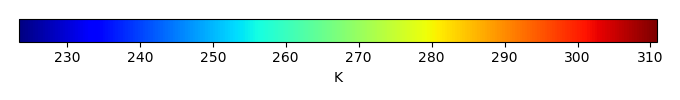MODEL MEANBIAS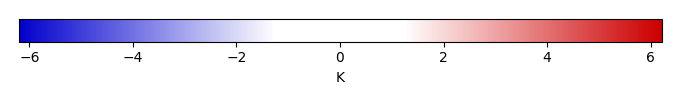BIAS SCORERMSE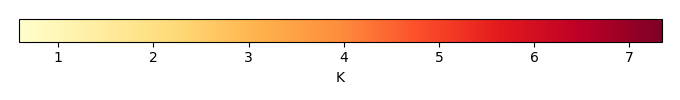RMSE SCOREBENCHMARK INTERANNUAL VARIABILITY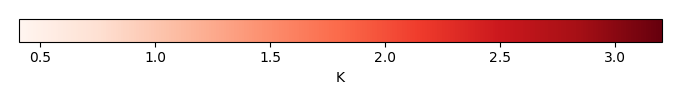MODEL INTERANNUAL VARIABILITYINTERANNUAL VARIABILITY SCOREBENCHMARK MAX MONTHMODEL MAX MONTHDIFFERENCE IN MAX MONTH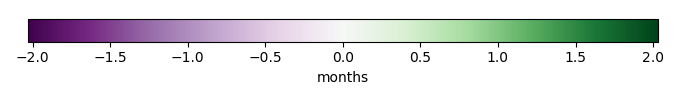SEASONAL CYCLE SCORESPATIAL TAYLOR DIAGRAMMODEL COLORS# Spatially integrated regional mean

MODEL COLORSREGIONAL MEANANNUAL CYCLEMONTHLY ANOMALYANNUAL CYCLE# All Models

BenchmarkCLM4CLM4.5CLM5# Data Information

creation_date: Fri Feb 8 14:12:14 PST 2019

history: 2018-01-15: download from https://crudata.uea.ac.uk/cru/data/hrg/cru_ts_4.01/cruts.1709081022.v4.01/ 2019-02-06: converted and created to netCDF4 for ILAMB package

original_resolution: 0.5x0.5

resolution: 0.5x0.5

original_frequency: mon

frequency: mon

references: Harris et al., 2014, Int. J. Climatol., doi:10.1002/joc.3711

institution: Original Data held at British Atmospheric Data Centre, RAL, UK.

source: Run ID = 1709081022. Data generated from:TMX derived from gridded TMP & DTR absolutes, DTR in turn derived from tmn.1708171135.dtb, tmx.1708171215.dtb

title: CRU TS4.01 Maximum Temperature

Conventions: CF-1.7